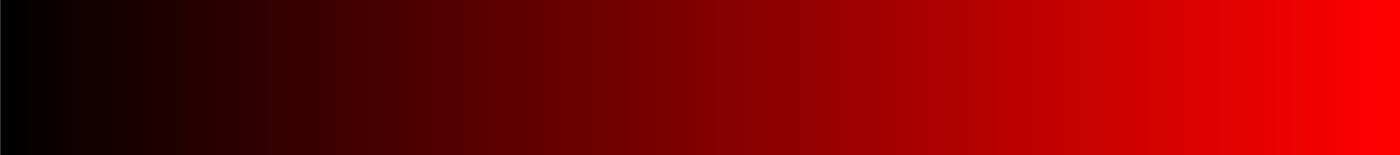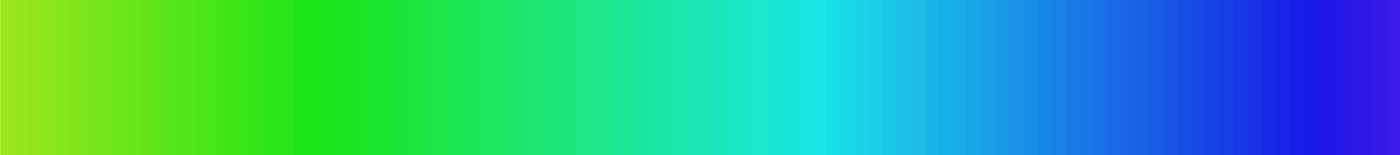# Randomness in CSS

The majority of the languages have some mechanisms for generating random numbers. Unfortunately, that is not the case in CSS. This might not be a problem for most websites, but when dealing with a more generative approach (which CSS is really great for) we have to resort to JavaScript to generate random values. In his article, I will explore a nice way of generating it using only CSS.

I will cover the following topics:

• Generating random numbers in CSS
• Using random values to generate ranges of numbers and colours
• Generating effect like in the cover for this article

# Random number generator functions

To generate random values in CSS we need first to understand how the randomness in computers works. Computers cannot come up with a random number by themselves — they were designed to work in a very rigorous way — for a given input they return a set output. All they can do is generate pseudo-random numbers — the sequence of numbers that looks like it’s random but which still has some underlying rules behind it.

An example of it might be the following function:

`f(x) = (A*x + c) mod 1;`

`A`and `c` parameters can be adjusted to our liking. `mod 1` is a notation meaning that we want to take a non-integer reminder of the number, for example `5.3 mod 1 = 0.3`.

The function will always give us results within the range of `0-1` which is handy because we can later scale it however we want without needing to change the function itself - when generating colours we will use different ranges when generating sizes in pixels.

Let’s set `A=8.385, c=2.534` then we will get the following pseudo-random sequence:

`fn(0)  = 0.5339999999999998fn(1)  = 0.9190000000000005fn(2)  = 0.3039999999999985fn(3)  = 0.6890000000000001fn(4)  = 0.07399999999999807fn(5)  = 0.4589999999999961fn(6)  = 0.8440000000000012fn(7)  = 0.2289999999999992fn(8)  = 0.6140000000000043fn(9)  = 0.9990000000000094fn(10) = 0.38400000000000034`

If you don’t know the underlying sequence the numbers generated by the function might look random.

In this case, given enough values, it is easy to reverse engineer the function, but the real-life random functions are usually much more complex. You can use higher order polynomials, trigonometry function and whatever else you imagine… unless you are working in plain CSS.

In CSS we do not have a big range of mathematical functions to operate on but we can still use some basic operations like sum and multiplication, and using `@property` we can perform floor, but there’s not much more than that. Fortunately, that’s more than enough to get satisfactory results.

# Our CSS Random Generator

In our CSS random number generator, we will use the same technique but, to make the function less predictable, we will use a second-degree polynomial — meaning we will include an extra component of `B*x^2`.

Moreover, our example will use sequential numbers to generate our random numbers. CSS does not natively offer us a mechanism to easily get the sequential numbers for each of the elements but, using the technique from my last article, we can generate it. The number will be stored as a `--n` CSS variable. For more information check out my previous article.

Here’s the solution for our random number generator:

The CodePen above plots the results of the function for the first 100 values.

The code uses `primeCounter` from my previous article to enumerate all divs (set as `--n` variable). This variable is then used to calculate `x0` - this is our random generation function. The values can grow extremely big though so we need to clamp them. To get only the non-integer part of it, we can use the technique described in this incredible CSS Tricks article - by defining `@property` of the type `<integer>` we can use it to floor the number - meaning that `5.35` will become `5`. By subtracting it from the original number we can finally get our result `mod 1`.

Now we can use it to set some parameters of our styles.

## Compatibility

The solution relies on `@property` to perform floor operation. It is not currently available in Firefox and Safari at the time of writing.

# Styling using random values

All the random values we have generated are from the range of `0 to 1`. That allows us to scale them to desired range and unit:

## Converting to length (width, height, etc).

To convert the value to the length we need to do the following:

`width: calc(50px + var(--r) * 100px);`

This will convert our random values to 50px-150px range. In general, you can use the following equation to scale values from the `0-1` range to your desired `a-b` range:

`calc(a + var(--r) * (b-a));`

## Converting to colour

To convert our random value to colour we need to decide what colour representation we want to use. CSS supports multiple representations including:

• RGB: red, green and blue components
• HSL: hue, saturation and luminance components

There are other useful techniques like using `color()` function or LCH / LAB colour space but they are not widely adopted in browsers at the time of writing.

Once you select the colour representation you want to use you can use it to vary the values accordingly:

`background-color: rgb(calc(127 + var(--r) * 127, 0, 0);`Spectrum for RGB(x, 0, 0), x from 0 to 255.

The code above will convert our random value to colours from the half intensity of red to the full intensity of red.

We can also vary the saturation (or luminance) of the colour using HSL colour space:

`background-color: hsl(170, calc(var(--r) * 100%), 50%);`Spectrum for HSL(170, x, 50%). x from 0% to 100%.

You can also use HSL space to vary the colours in the range. To have your random colours vary from light green to dark blue, you can use the following formula:

`hsl(calc(80 + var(--r) * 170), 80%, 50%);`Spectrum for HSL(x, 80%, 50%), x from 80 to 250.

# Example: Generative Skyline

We can use the techniques above to generate the following skyline. JavaScript in this example is used only to provide a new seed when moving the mouse. All calculations are done in CSS.

# Example: Shapes Grid

The following example shows how you can apply this technique to vary multiple styles of elements at the same time. Each of the 4 sides of the border-radius and colour is determined based on the random values. Colours are generated from the range `hsl(x, 50%, 50%)` for `x varying from 150 to 240`.

To generate the border radiuses we use the same single random number but for each of the edges, we scale it by different factors and clamp it within 0 and 1. Because each of those variables was configured as an integer (using `@property`), we end up with either 0 or 1. That value is then multiplied by 50% making the border either 0% or 50%.

## Example: Memphis Style Art Generator

The last example is a Memphis Group design style pattern generator. The style was popular in the 80s and in the last years, it is making its comeback in art and pop culture. All random generation is done in CSS, JavaScript is used only to provide the ability to change seed or animate the seed change.

Do you see any use cases where CSS-only randomness might be useful for you? Maybe you know other solutions to achieve those effects? Let me know in the comments!

--

--